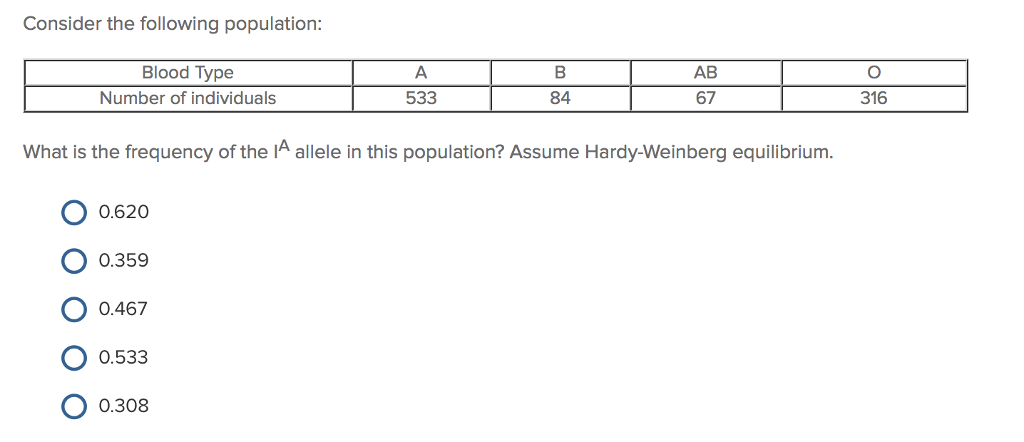# Problem: Consider the following population for the shown trait below. What is the frequency of the IA allele in this population? Assume Hardy-Weinberg equilibrium.A. 0.620B. 0.359C. 0.467D. 0.533E. 0.308

###### FREE Expert Solution

For the given trait (blood type) that follows Hardy-Weinberg equilibrium, the population is expected to follow the equation p2 + 2pq + q2 + 2qr + r2 + 2pr = 1, where p, q, and r are the alleles for the IA, IB, and i, respectively. From this equation, it can easily be deduced that r = 0.562, since the relative frequency for the blood type O phenotype, is 0.316.###### Problem Details

Consider the following population for the shown trait below. What is the frequency of the IA allele in this population? Assume Hardy-Weinberg equilibrium.

A. 0.620

B. 0.359

C. 0.467

D. 0.533

E. 0.308